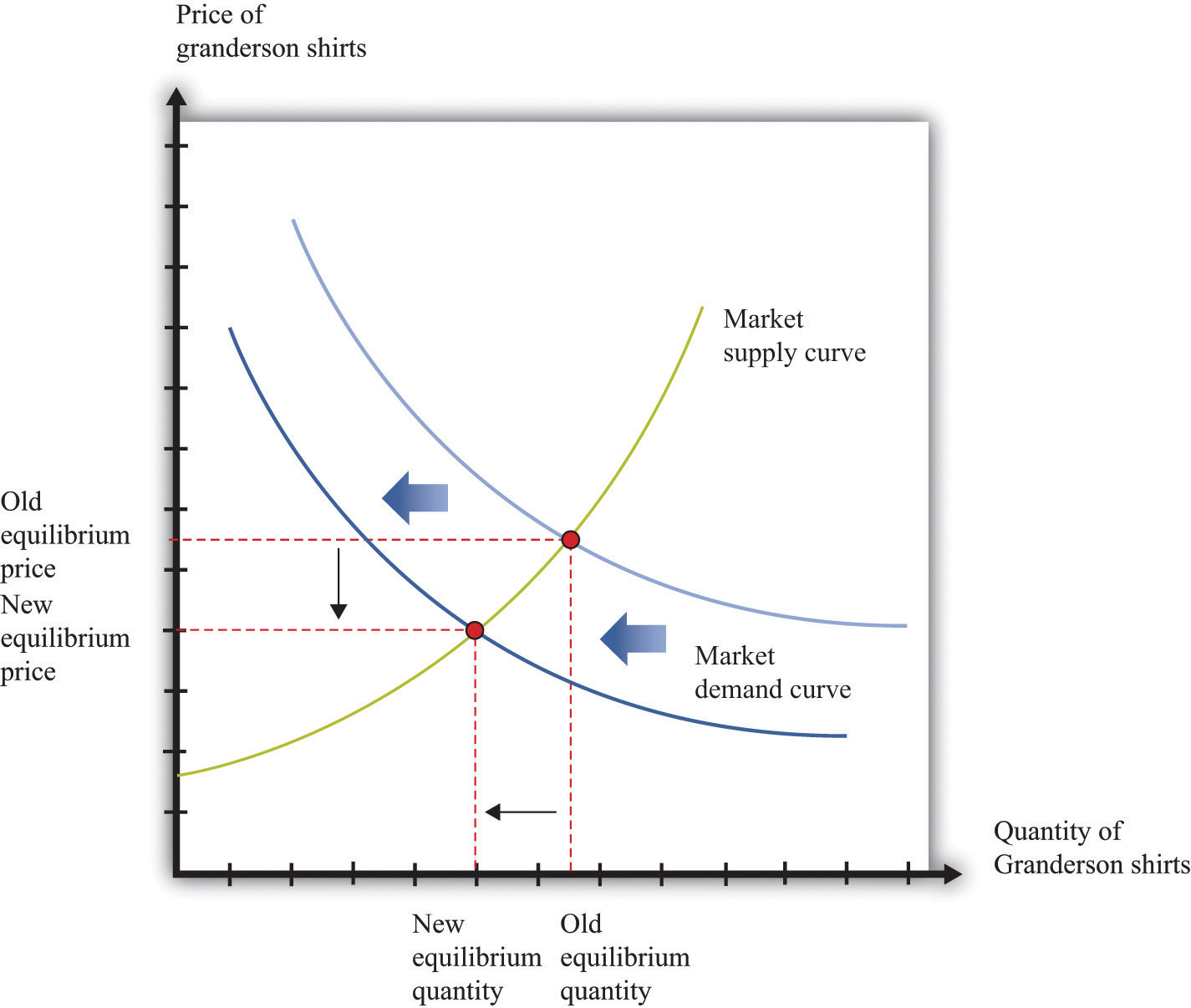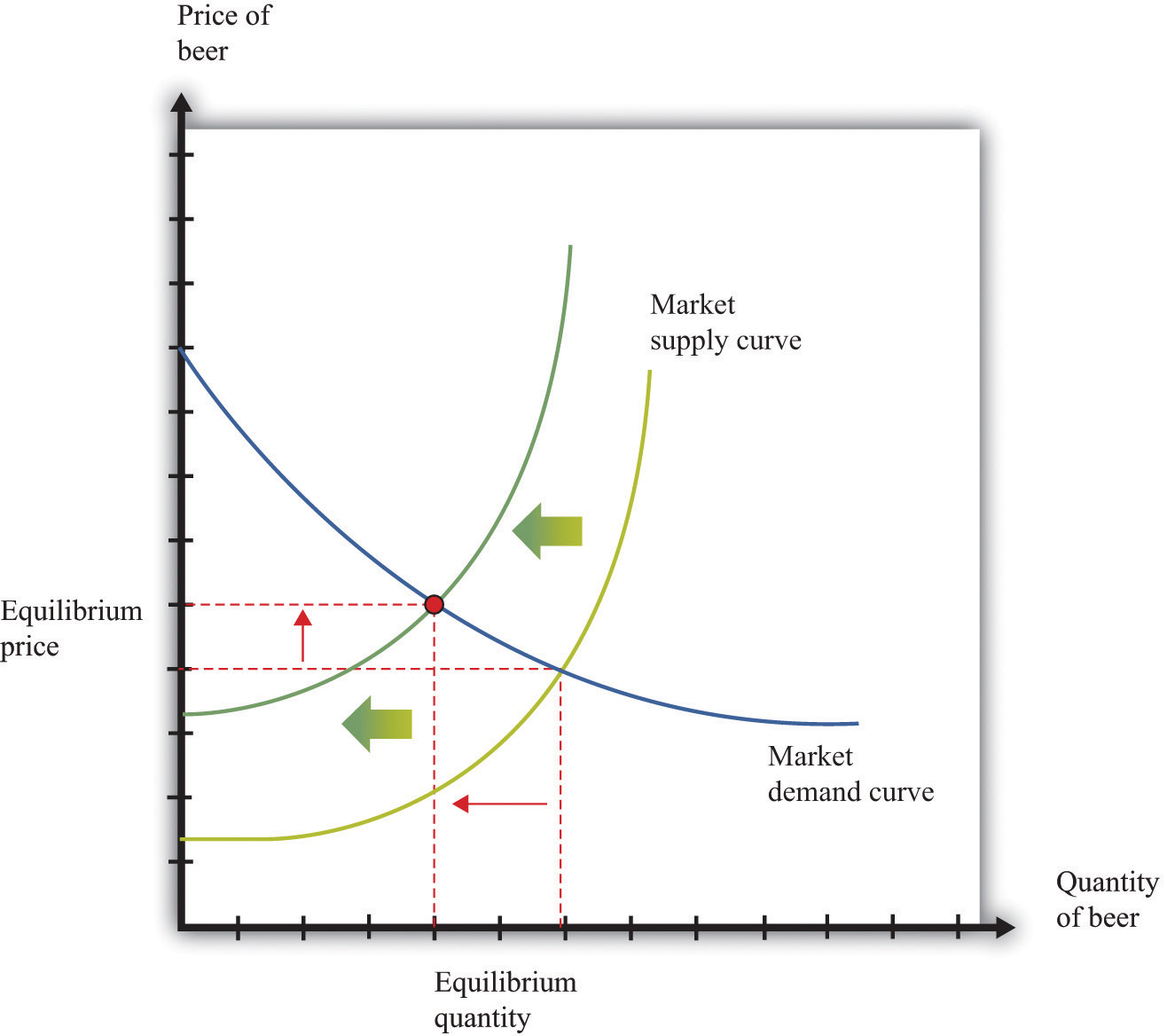## 17.16 Comparative Statics

Comparative statics is a tool used to predict the effects of exogenous variables on market outcomes. By exogenous variables, we mean anything that shifts either the market demand curve (for example, news about the health effects of consuming a product) or the market supply curve (for example, weather effects on a crop). By market outcomes, we mean the equilibrium price and the equilibrium quantity in a market. Comparative statics is a comparison of the market equilibrium before and after a change in an exogenous variable.

A comparative statics exercise consists of a sequence of five steps:

1. Begin at an equilibrium point where the quantity supplied equals the quantity demanded.
2. Based on the description of the event, determine whether the change in the exogenous factor shifts the market supply curve or the market demand curve.
3. Determine the direction of this shift.
4. After shifting the curve, find the new equilibrium point.
5. Compare the new and old equilibrium points to predict how the exogenous event affects the market.

Figure 17.13 "Shifts in the Demand Curve" and Figure 17.14 "Shifts in the Supply Curve" show comparative statics in action. In Figure 17.13 "Shifts in the Demand Curve", the market demand curve has shifted to the left. The consequence is that the equilibrium price and the equilibrium quantity both decrease. Notice that the demand curve shifts along a fixed supply curve. In Figure 17.14 "Shifts in the Supply Curve", an increase in the price of an input (hops) has shifted the market supply curve of beer to the left. The consequence is that the equilibrium price increases and the equilibrium quantity decreases. Notice that the supply curve shifts along a fixed demand curve.

## Key Insights

• Comparative statics is used to determine the market outcome when the market supply and demand curves are shifting.
• Comparative statics is a comparison of equilibrium points.
• If the market demand curve shifts, then the new and old equilibrium points lie on a fixed market supply curve.
• If the market supply curve shifts, then the new and old equilibrium points lie on a fixed market demand curve.

Figure 17.13 Shifts in the Demand CurveFigure 17.14 Shifts in the Supply Curve